• 归一化推导证明： 期望（一阶矩）推导证明： 二阶矩推导证明： 方差推导证明： 写在前面的唠叨： 最近这段时间一直在研究深度学习之类的东西，虽然如今对几种常见的神经网络都有了很好的了解，用起来也比较顺手...

目录

写在前面的唠叨：

归一化推导证明：

期望（一阶矩）推导证明：

二阶矩推导证明：

方差推导证明：

写在前面的唠叨：

最近这段时间一直在研究深度学习之类的东西，虽然如今对几种常见的神经网络都有了很好的了解，用起来也比较顺手，但是越学也越觉得瓶颈越来越明显了，最大的问题觉得还是数学基础不行，学习那些常见的模型已经把线性代数的知识捡的差不多了，而到了想自己设计模型的时候，才忽然发现微积分也是十分重要的，而这两年我都还给老师了呀T_T。所以把PRML这本书又翻了出来，推导一下里面的公式。

然而刚看到高斯分布里面的方差推导就抽了我一嘴巴，去网上查了查发现这部分推导大家写的都挺乱的，于是自己总结了一下，留作记录，省的以后在看的时候到处乱查，重新推……

归一化推导证明：

证明归一化，即证明：

首先我们将其展开：

这里将 替换掉有：

这里假设：

这个积分直接计算比较困难，但是可以绕个弯，采用极坐标的方式计算，首先我们将其求其平方：

在将其转化为极坐标，令可得：

即：

恩！大功告成！

期望（一阶矩）推导证明：

证明期望就比较简单了~

将 替换掉有：

这里为奇函数，所以该项积分为0，所以：

二阶矩推导证明：

就是这个东西，推了一上午都没推出来，最后还是的问了周围考研的同学，真是耻辱，被挂起来抽。这里给出了两种推导方式：

1. 展开利用Gamma函数进行求解：

将 替换掉有：

这里令第一项中，即有：

这里用到了函数，也就是我们通常说的伽马函数，它的定义是这样的：

这里有几个特殊值，感兴趣的话可以记一下。

特殊值内容来自中文wiki，所以出错了不负责哦，嘿嘿……

当然这里用到了伽马函数，也可以采用不用伽马函数的方法。

2. 直接积分求解：

啊~这个就是我同学的方法，实名感谢猛某，如果多年后您看见这篇博客别忘了朝我要稿费。

方差推导证明：

既然我们已经求的了一阶矩、二阶矩，那么再求方差就简单了：

当然方差也可以直接求，因为这部分已经有前辈推导过了，我就直接上图了！（才不是嫌麻烦，不想写latex代码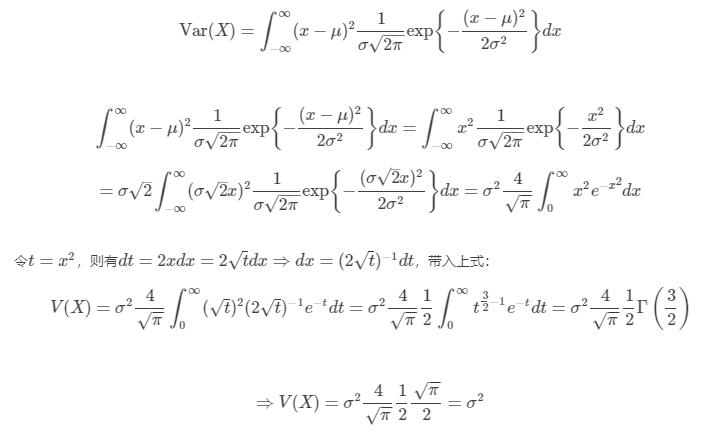这里标明出处，前辈的推导很棒，给了我很大帮助。

留个坑：

本来是想写个PRML的全公式推导的读书笔记，然而码完了这些代码，突然觉得好麻烦收获好大，尽力吧，如果时间充裕会补一补，留个坑，溜……

展开全文高斯分布 归一化
• 目录高斯变量基础高斯分布概率密度函数性质复高斯分布概率密度函数应用零均值循环对称复高斯随机变量零均值化卡方分布补充归一化标准化 高斯变量基础 高斯分布 概率密度函数 性质 复高斯分布 若复高斯分布Z=X+iY,...

高斯变量基础

高斯分布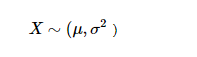概率密度函数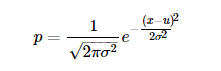性质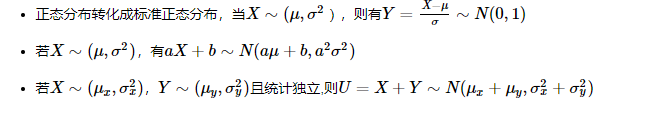复高斯分布

若复高斯分布Z=X+iY, 且满足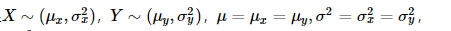则有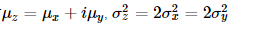概率密度函数注：复高斯随机变量的密度函数，分母已经没有根号

应用

零均值循环对称复高斯随机变量

特殊的，当μ=μx=μy=0时，Z称为零均值循环对称复高斯随机变量（zero mean circle symmetric complex gaussian,ZMCSCG）,σ2称为每个实数维度上的方差。

以上分析得出复高斯随机变量与每一实数维度高斯随机变量的关系

零均值化

将每个像素的值减去训练集上所有像素值的平均值，比如已计算得所有像素点的平均值为128，所以减去128后，现在的像素值域即为[-128,127]，即满足均值为零。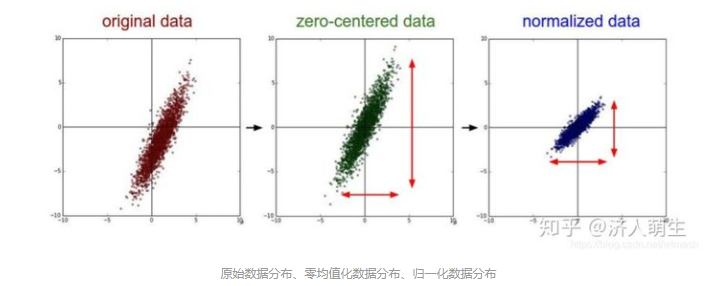卡方分布

设X1,X2,X3,…,i.i.d∼N(0,1), 令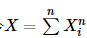, 则X是服从自由度为n的χ2分布，记为X∼χ2(n)
卡方分布为特殊的Gamma分布，服从参数为G(n/2,1/2)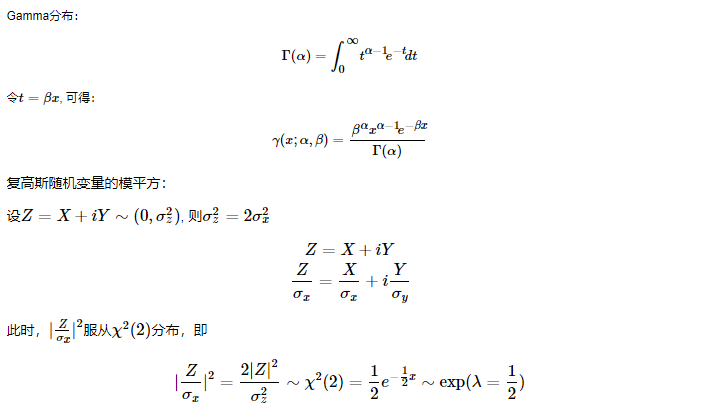注意：
复高斯随机变量概率表达式的分母
复高斯随机变量模平方的分布与卡方分布、指数分布、Gamma分布之间的关系

补充

归一化、标准化、零均值化核心思想：平移+缩放

归一化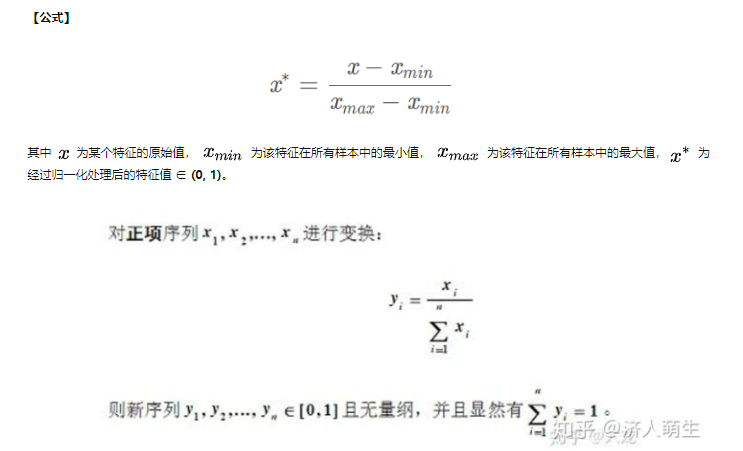【作用】将某个特征的值映射到[0,1]之间，消除量纲对最终结果的影响，使不同的特征具有可比性，使得原本可能分布相差较大的特征对模型有相同权重的影响，提升模型的收敛速度，深度学习中数据归一化可以防止模型梯度爆炸。

标准化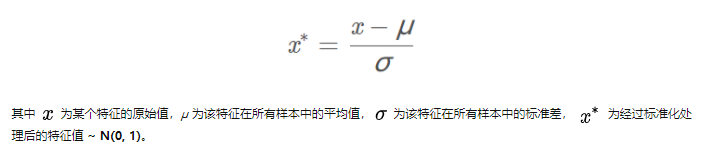【作用】将原值减去均值后除以标准差，使得得到的特征满足均值为0，标准差为1的正态分布，使得原本可能分布相差较大的特征对模型有相同权重的影响。举个例子，在KNN中，需要计算待分类点与所有实例点的距离。假设每个实例（instance）由n个features构成。如果选用的距离度量为欧式距离，数据预先没有经过归一化，那些绝对值大的features在欧式距离计算的时候起了决定性作用。

从经验上说，标准化后，让不同维度之间的特征在数值上有一定比较性，得出的参数值的大小可以反应出不同特征对样本label的贡献度，可以大大提高分类器的准确性。

【二者比较】具体应该选择归一化还是标准化呢，如果把所有维度的变量一视同仁，

①在计算距离中发挥相同的作用，应该选择标准化，标准化更适合现代嘈杂大数据场景。

②如果想保留原始数据中由标准差所反映的潜在权重关系，或数据不符合正态分布时，选择归一化。

参考https://zhuanlan.zhihu.com/p/183591302
https://www.cnblogs.com/zwwangssd/p/12540280.html

加性高斯白噪声

高斯是指概率分布是正态函数，白噪声是指他的二阶矩不相关，一阶矩为常数，是指先后信号在时间上的相关性。高斯白噪声是研究信道加性噪声的理想模型，通信中的主要噪声源—热噪声就属于这类噪声。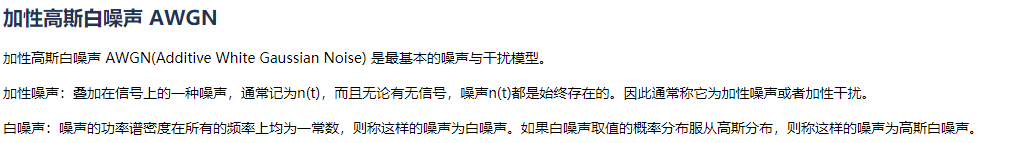展开全文• 我试图从文本文件中读取数据集,提取3个主要参数,并将它们放在单独的列表中,并在分配高斯分布函数后对参数列表(a,b,c)应用规范。为了取得好成绩我把正数和负数分开每个参数的列表并对其应用高斯分布函数分别地挑选:...

我试图从文本文件中读取数据集,提取3个主要参数,并将它们放在单独的列表中,并在分配高斯分布函数后对参数列表(a,b,c)应用规范化。为了取得好成绩我

把正数和负数分开

每个参数的列表并对其应用高斯分布函数

分别地

挑选:

意思是

价值

负数

作为真实

最小值

意思是

价值

正数

作为真实

最大值

而不是直接在这些参数的主列表中找到可能重复的最小最大值

几次

因为他们不合意

置信区间

. 如下图所示,所有步骤可分为4步完成,其中包括2个24x20矩阵图,图中数据帧中的一列由于数据的巨大回归和散射以及不必要的噪声(使其无法解释)而不清晰: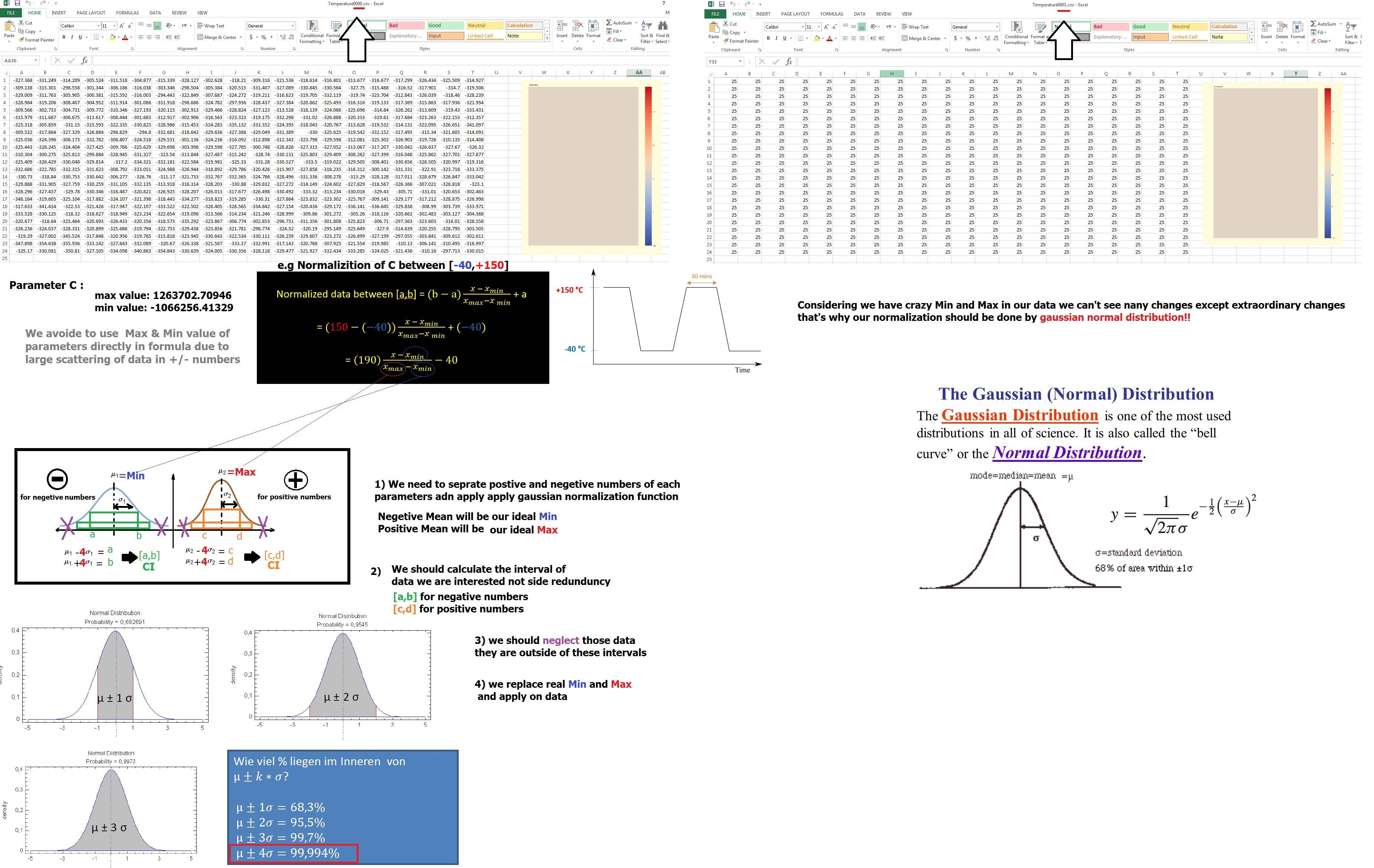考虑到像[a,b]这样的区间之间的标准化没有确定的答案,我根据它的公式定义函数:

def normalize(value, min_value, max_value, min_norm, max_norm):

new_value = ((max_norm - min_norm)*((value - min_value)/(max_value - min_value))) + min_norm

return new_value

所以我的剧本如下:

import numpy as np

import pandas as pd

import matplotlib.pyplot as plt

import scipy

import warnings

warnings.filterwarnings("ignore",category =RuntimeWarning)

id_set = dft[dft.index % 4 == 0].astype('int').values

A = dft[dft.index % 4 == 1].values

B = dft[dtf.index % 4 == 2].values

C = dft[dft.index % 4 == 3].values

data = {'A': A[:,0], 'B': B[:,0], 'C': C[:,0]} # arrays

#main_data contains all the data

df = pd.DataFrame(data, columns=['A','B','C'], index = id_set[:,0])

df = df.replace([np.inf, -np.inf], np.nan).astype(np.float64)

df = df.fillna(0.012345)

def normalize(value, min_value, max_value, min_norm, max_norm):

new_value = ((max_norm - min_norm)*((value - min_value)/(max_value - min_value))) + min_norm

return new_value

def createpositiveandnegativelist(listtocreate):

l_negative = []

l_positive = []

for value in listtocreate:

if (value < 0):

l_negative.append(value)

elif (value > 0):

l_positive.append(value)

#print(t_negative)

#print(t_positive)

return l_negative,l_positive

def calculatemean(listtocalculate):

return sum(listtocalculate)/len(listtocalculate)

def plotgaussianfunction(mu,sigma):

s = np.random.normal(mu, sigma,1000)

abs(mu - np.mean(s))<0.01

abs(sigma - np.std(s,ddof=1))<0.01

#count, bins, ignored = plt.hist(s,30,density=True)

#plt.plot(bins, 1/(sigma * np.sqrt(2 * np.pi)) * np.exp(-(bins-mu)**2/(2*sigma**2)),linewidth=2, color= 'r')

#plt.show()

return

def plotboundedCI(s, mu, sigma, lists):

plt.figure()

count, bins, ignored = plt.hist(s,30,density=True)

plt.plot(bins, 1/(sigma * np.sqrt(2 * np.pi)) * np.exp(-(bins-mu)**2/(2*sigma**2)),linewidth=2, color= 'r')

#confidential interval calculation

ci = scipy.stats.norm.interval(0.68, loc = mu, scale = sigma)

#confidence interval for left line

one_x12, one_y12 = [ci,ci], [0,3]

#confidence interval for right line

two_x12, two_y12 = [ci,ci], [0,3]

plt.title("Gaussian 68% Confidence Interval", fontsize=12, color='black', loc='left', style='italic')

plt.plot(one_x12, one_y12, two_x12, two_y12, marker = 'o')

plt.show()

#get sure about avoiding the outliers of CI

results = []

for value in lists:

if(ci< value

results.append(value)

else:

#print("NOT WANTED: ",value)

pass

return results

c_negative, c_positive = createpositiveandnegativelist(C)

b_negative, b_positive = createpositiveandnegativelist(B)

a_negative, a_positive = createpositiveandnegativelist(A)

#get max and min values

a_min = main_data['A'].min()

a_max = main_data['A'].max()

b_min = main_data['B'].min()

b_max = main_data['B'].max()

c_min = main_data['C'].min()

c_max = main_data['C'].max()

print ("\ntmp Negative Min",c_min)

print ("\n tmp Positive Max",c_max)

#calculating the mean value

c_p_mean = calculatemean(c_positive)

b_p_mean = calculatemean(b_positive)

a_p_mean = calculatemean(a_positive)

c_n_mean = calculatemean(c_negative)

b_n_mean = calculatemean(b_negative)

a_n_mean = calculatemean(a_negative)

print ("\ntmp Negative Mean",c_n_mean)

print ("\n tmp Positive Mean",c_p_mean)

#calculating the sigma value

c_sigma_Negative = np.std(c_negative)

c_sigma_Positive = np.std(c_positive)

b_sigma_Negative = np.std(c_negative)

b_sigma_Positive = np.std(c_positive)

a_sigma_Negative = np.std(c_negative)

a_sigma_Positive = np.std(c_positive)

#plot the gaussian function with histograms

plotgaussianfunction(c_p_mean, c_sigma_Positive)

plotgaussianfunction(c_n_mean, c_sigma_Negative)

plotgaussianfunction(b_p_mean, b_sigma_Positive)

plotgaussianfunction(b_n_mean, b_sigma_Negative)

plotgaussianfunction(a_p_mean, a_sigma_Positive)

plotgaussianfunction(a_n_mean, a_sigma_Negative)

#normalization

c_p_s = np.random.normal(c_p_mean, c_sigma_Positive, 1000)

c_n_s = np.random.normal(c_n_mean, c_sigma_Negative, 1000)

b_p_s = np.random.normal(b_p_mean, b_sigma_Positive, 1000)

b_n_s = np.random.normal(b_n_mean, b_sigma_Negative, 1000)

a_p_s = np.random.normal(a_p_mean, a_sigma_Positive, 1000)

a_n_s = np.random.normal(a_n_mean, a_sigma_Negative, 1000)

#histograms minus the outliers

c_p_results = plotboundedCI(c_p_s, c_p_mean, c_sigma_Positive, c_positive)

c_n_results = plotboundedCI(c_n_s, c_n_mean, c_sigma_Negative, c_negative)

b_p_results = plotboundedCI(b_p_s, b_p_mean, b_sigma_Positive, b_positive)

b_n_results = plotboundedCI(b_n_s, b_n_mean, b_sigma_Negative, b_negative)

a_p_results = plotboundedCI(a_p_s, a_p_mean, a_sigma_Positive, a_positive)

a_n_results = plotboundedCI(a_n_s, a_n_mean, a_sigma_Negative, a_negative)

#next iteration create all plots, change the number of cycles

for i in df:

if 'C' in i:

min_nor = -40

max_nor = 150

#Applying normalization for C between [-40,+150]

new_value3 = normalize(df['C'].iloc[j:j+480], c_n_mean, c_p_mean, -40, 150)

n_cbar_kws = {"ticks":[-40,150,-20,0,25,50,75,100,125]}

df3 = print_df(mkdf(new_value3))

else:

#Applying normalizayion for A,B between [-1,+1]

new_value1 = normalize(df['A'].iloc[j:j+480], a_n_mean, a_p_mean, -1, 1)

new_value2 = normalize(df['B'].iloc[j:j+480], b_n_mean, b_p_mean, -1, 1)

n_cbar_kws = {"ticks":[-1.0,-0.75,-0.50,-0.25,0.00,0.25,0.50,0.75,1.0]}

df1 = print_df(mkdf(new_value1))

df2 = print_df(mkdf(new_value2))

'''

for i in df:

if i=='C':

#Applying normalizayion for C between [-40,+150]

data['C'] = normalize(df[i].values, c_n_mean, c_p_mean, -40, 150)

elif i=='A':

#Applying normalization for A , B between [-1,+1]

data['A'] = normalize(df[i].values, a_n_mean, a_p_mean, -1, 1)

else:

data['B'] = normalize(df[i].values, b_n_mean, b_p_mean, -1, 1)

'''

norm_data = pd.DataFrame(data, index = id_set[:,0])

print(norm_data)

norm_data.to_csv('norm.csv')

问题是:

γ

问题I:

我已经避免了运行时警告错误,但仍然有以下错误,我不知道如何解决,包括

值错误:缩放<0

可能是由于正负数的分离。

γ

问题二:

在标准化之后,可能会发生超过10个周期(每个周期有480个值),我已经意识到考虑对两个-/+数字应用高斯函数,然后在[a,b]之间使用最小、最大的标准化,在这种情况下,仍有一些值超出范围,如下图所示或者列'c'超出了[-40,+150],这太奇怪了,超出了我的预期!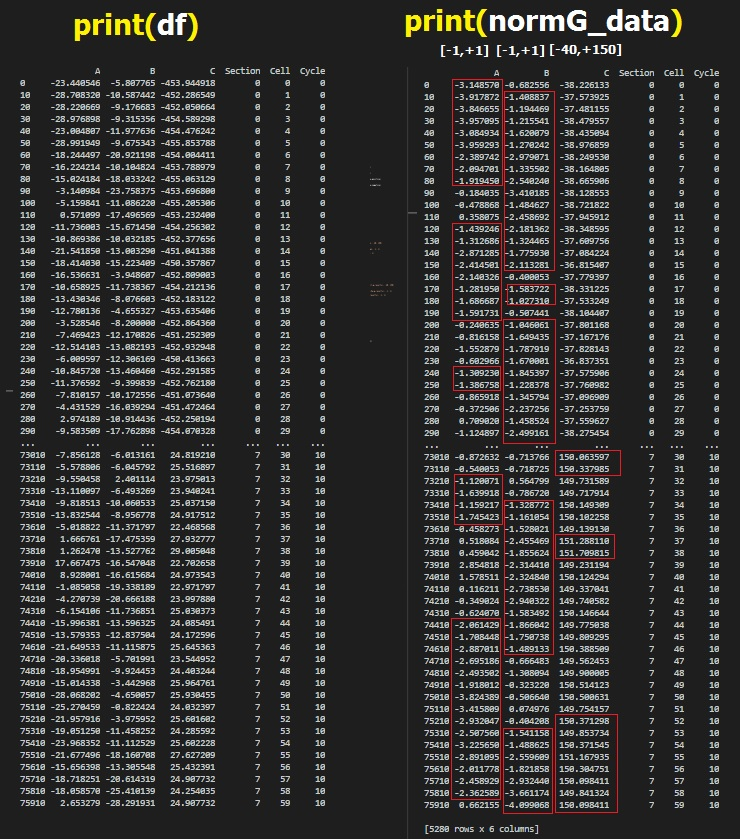希望有人对错误的解决方案有一个好的想法,或者使用高斯分布函数应用规范化的更好方法,谢谢您的关注。

注1:

我有一些

缺少数据(NAN或INF)

在我的值列表中,这些值已经被0替换了(确切地说是0.012345)!但是考虑到当我的参数列表中没有丢失的值时,代码就可以工作了!

注2:

我面临着这样的错误:

ValueError: max must be larger than min in range parameter.

对于

count, bins, ignored = plt.hist(s,30,density=True)

plotgaussianfunction(t_p_mean, t_sigma_Positive)

我认为是关于这个条件的:

abs(sigma - np.std(s,ddof=1)) < 0.01

因为我也有类似的错误

ValueError: scale < 0

对于

s = np.random.normal(mu, np.abs(sigma) ,1000)

我已经问过了

here

我提供了3个周期的数据集样本:

dataset

我还提供了11个周期的另一个数据集:

dataset

展开全文正负数据如何归一化
• 论文中遇到很重要的一个元素就是高斯核函数，但是必须要分析出高斯函数的各种潜在属性，本文首先参考相关材料给出高斯核函数的基础，然后使用matlab自动保存不同参数下的高斯核函数的变化gif动图，同时分享出源代码...

摘要

论文中遇到很重要的一个元素就是高斯核函数，但是必须要分析出高斯函数的各种潜在属性，本文首先参考相关材料给出高斯核函数的基础，然后使用matlab自动保存不同参数下的高斯核函数的变化gif动图，同时分享出源代码，这样也便于后续的论文写作。

高斯函数的基础

2.1 一维高斯函数

高斯函数，Gaussian Function， 也简称为Gaussian，一维形式如下：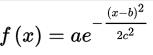对于任意的实数a,b,c，是以著名数学家Carl Friedrich Gauss的名字命名的。高斯的一维图是特征对称“bell curve”形状，a是曲线尖峰的高度，b是尖峰中心的坐标，c称为标准方差，表征的是bell钟状的宽度。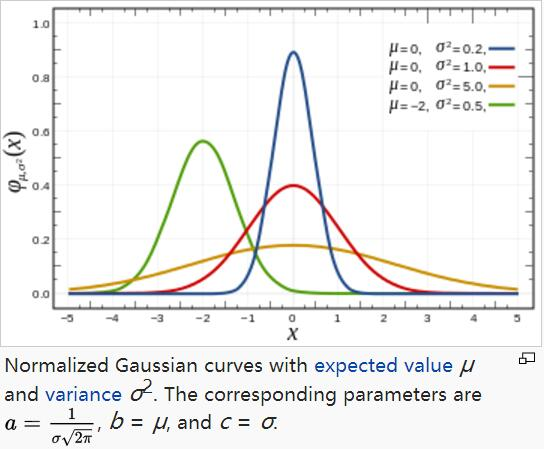高斯函数广泛应用于统计学领域，用于表述正态分布，在信号处理领域，用于定义高斯滤波器，在图像处理领域，二维高斯核函数常用于高斯模糊Gaussian Blur，在数学领域，主要是用于解决热力方程和扩散方程，以及定义Weiertrass Transform。

从上图可以看出，高斯函数是一个指数函数，其log函数是对数凹二次函数 whose logarithm a concave quadratic function。

高斯函数的积分是误差函数error function，尽管如此，其在整个实线上的反常积分能够被精确的计算出来，使用如下的高斯积分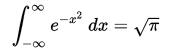同理可得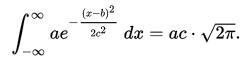当且仅当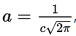上式积分为1，在这种情况下，高斯是正态分布随机变量的概率密度函数，期望值μ=b，方差delta^2 = c^2，即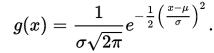2.2 二维高斯函数

二维高斯函数，形如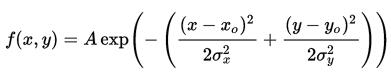A是幅值，x。y。是中心点坐标，σσy是方差，图示如下，A = 1, xo = 0, yo = 0, σx = σy = 1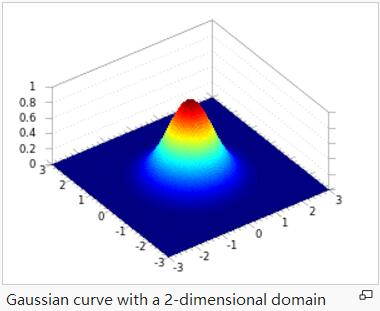2.3 高斯函数分析

这一节使用matlab直观的查看高斯函数，在实际编程应用中，高斯函数中的参数有

ksize 高斯函数的大小

sigma 高斯函数的方差

center 高斯函数尖峰中心点坐标

bias 高斯函数尖峰中心点的偏移量，用于控制截断高斯函数

为了方便直观的观察高斯函数参数改变而结果也不一样，下面的代码实现了参数的自动递增，并且将所有的结果图保存为gif图像，首先贴出完整代码：

function mainfunc()
% 测试高斯函数，递增的方法实现高斯函数参数的改变对整个高斯函数的影响，
% 并自动保存为gif格式输出。
% created by zhao.buaa 2016.09.28

%% 保存gif动画
item = 10;      % 迭代次数
dt = 1;             % 步长大小
ksize =20;      % 高斯大小
sigma = 2;      % 方差大小
% filename = ['ksize-' num2str(ksize) '--' num2str(ksize+dt*item) '-sigma-' num2str(sigma) '.gif']; %必须预先建立gif文件
filename = ['ksize-' num2str(ksize)  '-sigma-' num2str(sigma) '--' num2str(sigma+dt*item) '.gif']; %必须预先建立gif文件

% main loop
for i = 1:item
center  = round(ksize/2);          % 中心点
bias       = ksize*10/10;              % 偏移中心点量
ksigma = ksigma(ksize, sigma, center, bias);    % 构建核函数
tname  = ['ksize-' num2str(ksize) '-sigma-' num2str(sigma) '-center-' num2str(center)];
figure(i), mesh(ksigma), title(tname);
%设置固定的x-y-z坐标范围，便于观察，axis([xmin xmax ymin ymax zmin zmax])
axis([0 ksize 0 ksize 0 0.008]);  view([0, 90]);% 改变可视角度
% ksize 递增
%     ksize = ksize + 10;
% sigma 递增
sigma = sigma + dt;

% 自动保存为gif图像
frame = getframe(i);
im = frame2im(frame);
[I,map] = rgb2ind(im,256);
if i==1
imwrite(I,map,filename,'gif','Loopcount',inf, 'DelayTime',0.4);
else
imwrite(I,map,filename,'gif','WriteMode','append','DelayTime',0.4);
end
end;

close all;

%% 截断高斯核函数，截断的程度取决于参数bias
function ksigma = ksigma(ksize, sigma, center,bias)
%ksize = 80;    sigma = 15;
ksigma=fspecial('gaussian',ksize, sigma);   % 构建高斯函数
[m, n] =size(ksigma);
for i = 1:m
for j = 1:n
if(  (i<center-bias)||(i>center+bias)||(j<center-bias)||(j>center+bias)  )
ksigma(i,j) = 0;
end;
end;
end;

结果图：

固定ksize为20，sigma从1-9，固定center在高斯中间，并且bias偏移量为整个半径，即原始高斯函数。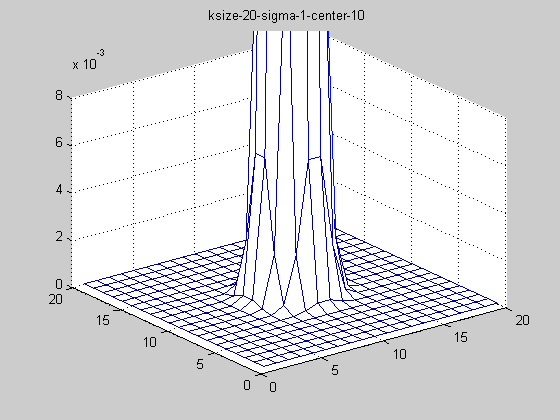随着sigma的增大，整个高斯函数的尖峰逐渐减小，整体也变的更加平缓，则对图像的平滑效果越来越明显。

保持参数不变，对上述高斯函数进行截断，即truncated gaussian function，bias的大小为ksize*3/10，则结果如下图：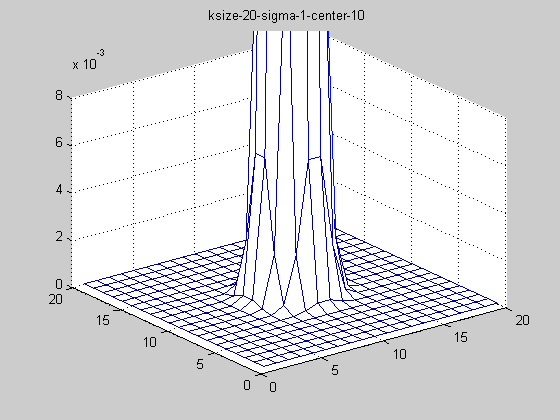truncated gaussian function的作用主要是对超过一定区域的原始图像信息不再考虑，这就保证在更加合理的利用靠近高斯函数中心点的周围像素，同时还可以改变高斯函数的中心坐标，如下图：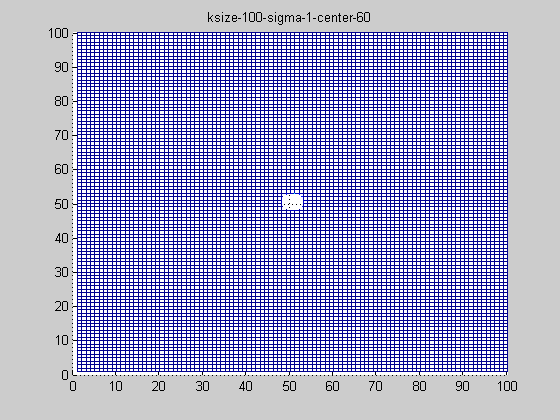为了便于观察截断的效果，改变了可视角度。

高斯核函数卷积

论文中使用gaussian与feature map做卷积，目前的结果来看，要做到随着到边界的距离改变高斯函数的截断参数，因为图像的边缘如果使用原始高斯函数，就会在边界地方出现特别低的一圈，原因也很简单，高斯函数在与原始图像进行高斯卷积的时候，图像边缘外为0计算的，那么如何解决边缘问题呢？

先看一段代码：

% 截断高斯核函数
ksize = 40; ksigma=fspecial('gaussian',  ksize, 6);
[m, n] =size(ksigma);
for i = 1:m
for j = 1:n
if( i<25 )
ksigma(i,j) = 0;
end;
end;
end;
figure, mesh(ksigma);

在i，即row上对高斯核函数进行截断，bias为半径大小，则如图6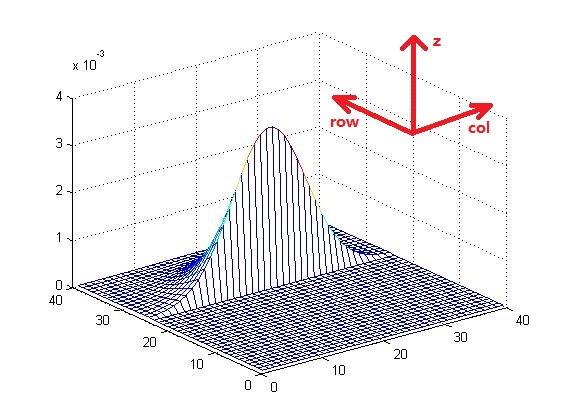并且对下图7进行卷积，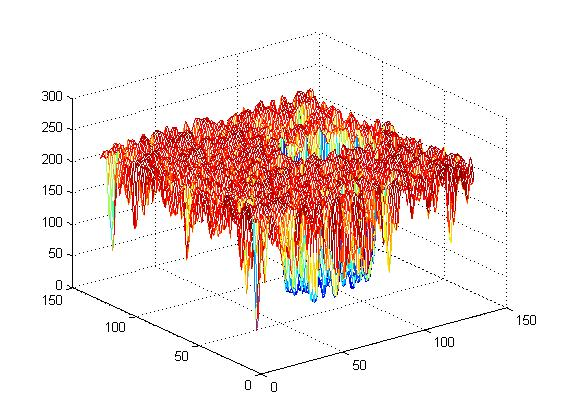首先卷积核为原始未截断高斯核函数，则结果如图8可以看出，在图像边缘处的卷积结果出现不想预见的结果，边缘处的值出现大幅度减少的情况，这是高斯核函数在边缘处将图像外的部分当成0计算的结果，因此，需要对高斯核函数进行针对性的截断处理，但是前提是要掌握bias的规律，下面就详细分析。

如果使用图6的高斯核函数与图7做卷积操作，则如图9：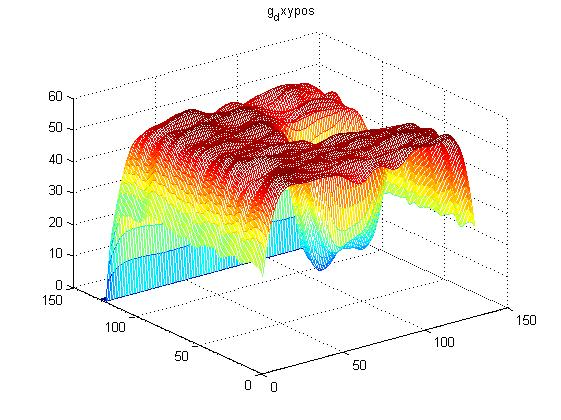可以看出，相比较于图8，与高斯核函数相对应的部分出现了变化，也就是说：

if( i<25 )
ksigma(i,j) = 0;
end;

靠近边缘的时候，改变 i 或 j 的值，即可保证边缘处的平滑处理。但是这样改变高斯核函数，使用matlab不是很好解决这个问题，还是使用将待处理图像边缘向外部扩展bias的大小，与标准高斯核函数做卷积，再将超过原始图像大小的部分剪切掉，目前来看在使用matlab中imfilter函数做卷积运算最合适且最简单的处理方法了，先写在这里，此部分并不是论文中的核心部分，只是数值运算的技巧性编程方法。

设计图像处理

深入地探讨高斯滤波与图像处理的关联，包括参数的影响、参数的选取、高斯模板的形成以及自行编程实现高斯滤波的效果与openCV函数实现效果比对。

说道“sigma表示的是标准差，如果标准差比较小，这是就相当于图像点运算，则平滑效果不明显；反之，标准差比较大，则相当于平均模板，比较模糊”，那么这么说可能很多人包括一开始的我并不是很理解，这是为什么呢，那么我们需要从高斯函数谈起：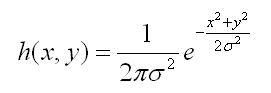这样一个高斯函数的概率分布密度如下图所示：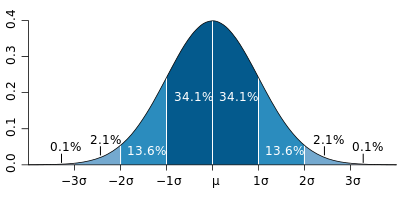我们要理解好这个图，横轴表示可能得取值x，竖轴表示概率分布密度F(x)，那么不难理解这样一个曲线与x轴围成的图形面积为1。sigma（标准差）决定了这个图形的宽度，我给出下述结论：sigma越大，则图形越宽，尖峰越小，图形较为平缓；sigma越小，则图形越窄，越集中，中间部分也就越尖，图形变化比较剧烈。这其实很好理解，如果sigma也就是标准差越大，则表示该密度分布一定比较分散，由于面积为1，于是尖峰部分减小，宽度越宽（分布越分散）；同理，当sigma越小时，说明密度分布较为集中，于是尖峰越尖，宽度越窄！

理解好上述结论之后，那么（一）中的结论当然也就顺理成章了，sigma越大，分布越分散，各部分比重差别不大，于是生成的模板各元素值差别不大，类似于平均模板；sigma越小，分布越集中，中间部分所占比重远远高于其他部分，反映到高斯模板上就是中心元素值远远大于其他元素值，于是自然而然就相当于中间值得点运算。

程序也可以验证如下：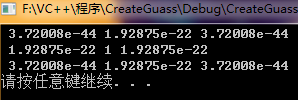窗口尺寸：3*3      sigma = 0.1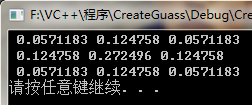窗口尺寸：3*3      sigma = 0.8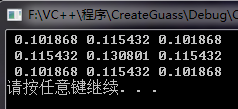窗口尺寸：3*3      sigma = 2

接着，我们来重点讨论下高斯模板，在初学高斯滤波的时候，用得最多的也是最经典的一个3*3模板就是

[1  2  1  ]

[ 2  4  2 ]

[1  2  1]

当时我就很纳闷这个模板是怎么出来的，后来我经过多方查找资料，基本得到了如下的解释：高斯模板实际上也就是模拟高斯函数的特征，具有对称性并且数值由中心向四周不断减小，这个模板刚好符合这样的特性，并且非常简单，容易被大家接受，于是就比较经典！但是这样一个简单的矩阵是远远不能满足我们对图像处理的要求的，我们需要按照自己的要求得到更加精确的模板，那么接下来我们就编程实现自己想要的高斯模板。部分关键函数如下：

double** createG(int iSize, double sigma)
{
double **guass;
double sum = 0;
double x2 = 0;
double y2 = 0;
int center = (iSize - 1) / 2;
guass = new double*[iSize];//注意，double*[k]表示一个有10个元素的指针数组

for (int i = 0; i < iSize; ++i)
{
guass[i] = new double[iSize];
}
for (int i = 0; i<iSize; i++)
{//使用x2，y2降低了运算速度，提高了程序的效率
x2 = pow(double(i - center), 2);
for (int j = 0; j<iSize; j++)
{
y2 = pow(double(j - center), 2);
sum += guass[i][j] = exp(-(x2 + y2) / (2 * sigma*sigma));
}
}
if (sum != 0)
{
//归一化
for (int i = 0; i<iSize; i++)
{
for (int j = 0; j<iSize; j++)
{
guass[i][j] /= sum;
}
}
}
return guass;
}

上述的这个函数就是返回的一个用户想要的高斯模板，其输入参数iSize表示模板大小（这里先只考虑方阵模板），输入参数sigma表示高斯函数标准差，聪明的你应该一眼就看出这个程序实际上就是按照公式根据规定的矩阵大小进行离散化得到相应的数据。纵观整个程序，我觉得最不好理解的是那个参数center，这是个什么参数呢？通过程序可以看出为什么center与iSize呈2center+1的关系呢？而且，为什么x2，y2是那样取值呢？这实际上是模拟的一种距离关系。举个例子来说：

假设我现在想要使用窗口尺寸为2k+1*2k+1的高斯模板对点进行操作，那么假设这个高斯模板的第一个元素地址记为为（1,1），那么高斯模板中心元素很容易算出为（k，k）。假设现在在计算模板中（i，j）元素值，那么公式中的x2模拟为该点到中心点的距离，即i-k-1（为什么要-1，读者不妨自己自己写个3*3的矩阵，看看（1,1）元素到（2,2）元素是不是要走两步）。但是程序中是没有-1的，这是因为程序中的i是从0开始的！于是这个center参数实际上就是代表的中心点！

运行结果：我的程序运行的结果：3*3，sigma=1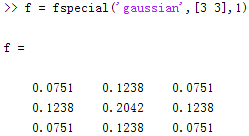Matlab的程序运行的结果：3*3，sigma=1

可以看出，相差无几，这个程序是成功的！

然后，最重要的部分当然是使用上述高斯模板对图像进行滤波处理得到想要的效果，那么接下来重点论述滤波处理。要理解好高斯滤波，自己写高斯滤波的算法当然是最好的，然后再和openCV的函数进行效果比对，这样，算是对高斯滤波有了比较好的认识和理解了！

在很多资料上，我们都看到高斯函数的这样一个特性，可分离性，意思是一个二维的高斯函数可以分解成相同的一维高斯函数处理两遍，得到的效果是一样的，但是处理时间却大大缩短了。

我们可以想到，二维函数直接处理会是这样的：

for(int i = 0; i < img->height; ++i)

for(int j = 0; j < img->weigth; ++j)

{

for(int m = 0; m < iSize; ++m)

for(int n= 0; n< iSize; ++n)

{

...

}

}

这样的算法复杂度可以看出是为O（height*weigth*iSize^2）

然而使用分解后的一维函数进行两次处理，程序应当如下：

for(int i = 0; i < img->height; ++i)

for(int j = 0; j < img->weigth; ++j)

{

for(int m = 0; m < iSize; ++m)

{

...

}

}

for(int j = 0; j< img->weigth; ++j)

for(int i= 0; i< img->height; ++i)

{

for(int m = 0; m < iSize; ++m)

{

...

}

}

这样的算法复杂度为O（2*height*weigth*iSize），比一维处理要少了很多，所以时间对应来说也会快一点。

用后者，我们的关键函数如下：

/*
生成一维高斯模板，水平的和垂直方向上的模板是一样的
输入参数分别是：模板大小，sigma值
输出：一维数组（高斯模板）
*/
double* CreateMuban(int iSize ,double sigma)
{
double *gauss = new double[iSize];//声明一维模板
int radius = (iSize - 1) / 2;//这是高斯半径
double MySigma = 2 * sigma * sigma;
double value = 0;
for (int i = 0; i < iSize; i++)
{//高斯函数前面的常数项因为在归一化的时候将会消去，故这里不重复计算
value = value + gauss[i];
}
for (int i = 0; i < iSize; i++)
{//归一化
gauss[i] = gauss[i] / value;
}
return gauss;
}

//对像素进行操作
IplImage*  operatorImage(IplImage* img, double* Muban, int iSize)
{
//创建一张新的图片来进行滤波操作
IplImage* NewImage = cvCreateImage(cvSize(img->width, img->height), 8, 3);
int radius = (iSize - 1) / 2;
int r = 0;
int g = 0;
int b = 0;
CvScalar cs;
//复制图片
cvCopy(img, NewImage);
//先对I，也就是垂直方向进行操作
for (int j = 0; j < NewImage->width; ++j)
{
for (int i = 0; i < NewImage->height; ++i)
{
//先判断是否是边缘,不是则操作，是则跳过不处理，保持原样
{
for (int k = 0; k < iSize; ++k)
{
/* b = b + (int)((double)(NewImage->imageData + (i - radius + k) * NewImage->widthStep)[j * (int)NewImage->nChannels + 0] * Muban[k]);
g = g + (int)((double)(NewImage->imageData + (i - radius + k) * NewImage->widthStep)[j * (int)NewImage->nChannels + 1] * Muban[k]);
r = r + (int)((double)(NewImage->imageData + (i - radius + k) * NewImage->widthStep)[j * (int)NewImage->nChannels + 2] * Muban[k]); */

cs = cvGet2D(NewImage, i - radius + k, j);   //获取像素
b = b + (int)((double)cs.val * Muban[k]);
g = g + (int)((double)cs.val * Muban[k]);
r = r + (int)((double)cs.val * Muban[k]);

}
/*((uchar *)(NewImage->imageData + i * NewImage->widthStep))[j * NewImage->nChannels + 0] = b;   //改变该像素B的颜色分量
((uchar *)(NewImage->imageData + i * NewImage->widthStep))[j * NewImage->nChannels + 1] = g;   //改变该像素G的颜色分量
((uchar *)(NewImage->imageData + i * NewImage->widthStep))[j * NewImage->nChannels + 2] = r;   //改变该像素R的颜色分量  */
cs = cvGet2D(NewImage, i, j);
cs.val = b;
cs.val = g;
cs.val = r;
cvSet2D(NewImage, i, j, cs);
b = 0;
g = 0;
r = 0;
}
}
}
//在对J，也就是水平方向进行操作
for (int i = 0; i < NewImage->height; ++i)
{
for (int j = 0; j < NewImage->width; ++j)
{
//先判断是否是边缘,不是则操作，是则跳过不处理，保持原样
{
for (int k = 0; k < iSize; ++k)
{
/*b = b + (int)((double)(NewImage->imageData + i * NewImage->widthStep)[(j - radius + k) * (int)NewImage->nChannels + 0] * Muban[k]);
g = g + (int)((double)(NewImage->imageData + i * NewImage->widthStep)[(j - radius + k) * (int)NewImage->nChannels + 1] * Muban[k]);
r = r + (int)((double)(NewImage->imageData + i * NewImage->widthStep)[(j - radius + k) * (int)NewImage->nChannels + 2] * Muban[k]);*/

cs = cvGet2D(NewImage, i, j - radius + k);   //获取像素
b = b + (int)((double)cs.val * Muban[k]);
g = g + (int)((double)cs.val * Muban[k]);
r = r + (int)((double)cs.val * Muban[k]);

}
/*((uchar *)(NewImage->imageData + i * NewImage->widthStep))[j * NewImage->nChannels + 0] = b;   //改变该像素B的颜色分量
((uchar *)(NewImage->imageData + i * NewImage->widthStep))[j * NewImage->nChannels + 1] = g;   //改变该像素G的颜色分量
((uchar *)(NewImage->imageData + i * NewImage->widthStep))[j * NewImage->nChannels + 2] = r;   //改变该像素R的颜色分量*/

cs = cvGet2D(NewImage, i, j);
cs.val = b;
cs.val = g;
cs.val = r;
cvSet2D(NewImage, i, j, cs);
b = 0;
g = 0;
r = 0;
//cout << r << " " << g << " " << b << endl;
}
}
}

return NewImage;
}

实现效果：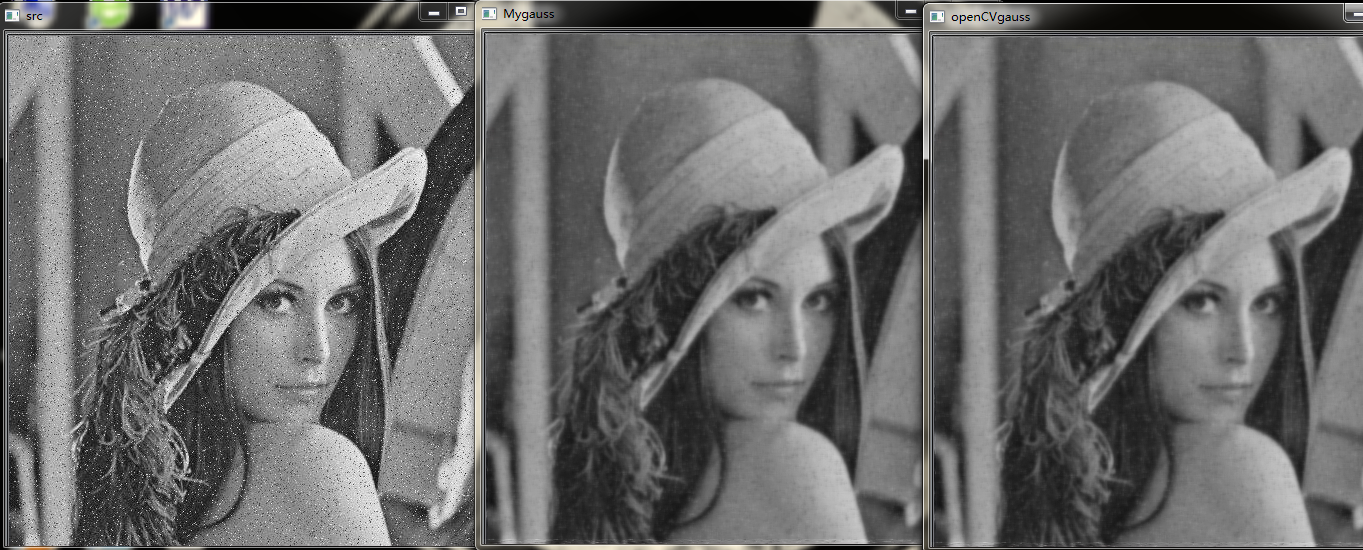自己编的程序与openCV函数处理效果并无差别，成功！

最后，对高斯滤波部分进行扩展------自适应高斯滤波。为了达到更好的滤波效果，我们的手法需要更加灵活，往往可以加上一些限制条件进行判断是否需要处理。这一点很想PID控制中的选择积分的方法（具体名字忘了···囧）。这个我将在后面进行适当地尝试！

学习高斯滤波只是一个开始，但是学习其他的图像处理方法诸如此类，我要学会举一反三，而且要实践与理论紧密结合，真正做到知其甚解才好啊！

展开全文• 高斯核函数

2019-06-27 22:51:08
#归一化 gaussKernel = gaussMatrix/sumGM return gaussKernel #高斯卷积核旋转180度不变，可以分离一维垂直和一维水平 #opencv提供了一维垂直高斯卷积核 cv2.getGaussianKernel(int ksize,double sigma,int ...高斯核函数
• 高斯函数半高宽

千次阅读 2021-05-05 03:12:21
设入射椭圆高斯光束光强分布方程为其中 ωx0 和ωy0 分别为椭圆高斯...对于高斯光束,其光强分布函数为 图 1 是运用 MATLAB 绘制的光束截面光强分布曲线,反映了 β 值对平顶光束 曲线形状影响。 图中高斯光束的束腰半...
• Matalb归一化函数

千次阅读 2014-04-18 15:22:29
线性归一化 一般可以这样写 function N = linear_normalize(D) % Linear Normalization max_value = max(max(D)); min_value = min(min(D)); N = (D-min_value) / (max_value-min_value); end 当然也可以归一...
• 高斯函数及高斯滤波器

万次阅读 多人点赞 2019-07-02 16:51:09
1 一维高斯分布 1.1 一维高斯分布的定义 若连续型随机变量X的概率密度为： 其中，为常数，则称X服从参数为，的正态分布或高斯分布，记为 1.2 一维高斯分布的曲线 横轴表示可能的取值x，竖轴表示...
• 可以实现正态分布（也就是高斯分布） pdf ——概率密度函数标准形式是： norm.pdf(x, loc, scale)等同于norm.pdf(y) / scale ，其中 y = (x - loc) / scale stats.norm主要公共方法如下： rvs:随机变量（就是从这个...python 数据挖掘 数据分析
• 有时候我们在拿到原始数据的时候，我们不能直接使用。大概场景有下面这些，我遇到的1....数据的标准化(normalization)和归一化数据的标准化(normalization)是将数据按比例缩放，使之落入一个小的特...
• 本代码为Python3.x，包括高斯分布及二维高斯分布代码，使用了numpy、scipy、matplotlib等包，适合初学者使用
• 函数为用户输入坐标和中心点、max/e 处的大小（宽度）和超高斯 P 值创建区域归一化高斯。 另一个函数显示了如何将噪声数据拟合到超高斯。 首先，估计面积、宽度和中心点。 然后找到可能的超高斯整数扫描的最小值...matlab
• 归一化方法总结

万次阅读 多人点赞 2015-10-05 20:21:27
Ref: http://blog.csdn.net/zbc1090549839/article/details/44103801 ... ============================== ...归一化方法（Normalization Method） 1。 把数变为（0，1）之间的小数 主要是为了数据处
• 归一化的方法及作用

万次阅读 多人点赞 2018-11-22 19:40:03
（一）归一化的作用 在机器学习领域中，不同评价指标（即特征向量中的不同特征就是所述的不同评价指标）往往具有不同的量纲和量纲单位，这样的情况会影响到数据分析的结果，为了消除指标之间的量纲影响，需要进行...归一化
• Matlab归一化方法

万次阅读 2018-05-10 10:41:09
1，标准归一化。 将原始数据集归一化为均值为0、方差1的数据集，归一化公式如下： x∗=x−μδ　其中μ为所有样本数据的均值，δ为所有样本数据的标准差。 这种方式要求原始数据集的分布近似为正态（高斯）分布。...
• 图像归一化是指对图像进行了一系列标准的处理变换，使之变换为一固定标准形式的过程，该标准图像称作归一化图像。 原始图像在经历一些处理或攻击后可以得到多种副本图像，这些图像在经过相同参数的图像归一化处理后...ArcGIS 归一化 NDVI 栅格计算器
• 这种回归方式称为高斯函数回归。依据不同形式的核函数，可以计算出不同的自变量样本权重。 期权的隐含波动率与期权的在值程度和期权剩余到期时间具有函数关系。本文用高斯函数回归的方式，选取2020年12月30日...
• 一、标准化和归一化的区别归一化其实就是标准化的一种方式，只不过归一化是将数据映了[0,1]这个区间中。标准化则是将数据按照比例缩放，使之放到一个特定区间中。标准化后的数据的均值＝0，标准差＝1，因而标准化的...
• 高斯函数与高斯滤波

千次阅读 2018-09-18 20:51:01
一维高斯函数我们都熟悉，形式如下： G(x)=12π−−√σexp(−x22σ2) G(x)=12πσexp⁡(−x22σ2) 计算机视觉中，高斯滤波使用的高斯核为xx和yy两个一维高斯的乘积，两个维度上的标准差σσ通常相同，形式如下： ...
• 重温归一化(MinMaxScaler)和标准化(StandardScaler)

万次阅读 多人点赞 2019-12-30 13:37:17
最近做一个时空序列预测的一个问题，用到了数据归一化和标准化，之前一直想花点时间看一下这俩的区别究竟是啥？ 现在参考了几篇博文，加上自己的一些理解，来具体的总结总结。 数据的归一化是无量纲化，也就是忽略...数据归一化 数据标准化 数据中心化
• 数据归一化的基本方法

千次阅读 2021-04-20 01:03:14
1.线性归一化简单公式表达：y = (x-min Value)/(max Value-min Value)其中，x是归一化之前的数据，y是归一化之后的数据，max Value 和 min Value 分别对应这一组数据中的最大值和最小值。范围：[0,1]。适用于：把...
• 支持向量机，高斯函数背景介绍核心思想公式推导及主要函数函数核心思想公式推导及函数名词介绍希尔伯特空间（Hilbert space）内积归一化和标准化内积空间（pre-Hilbert space/欧几里得空间）范数范数和距离...机器学习 支持向量机 深度学习
• 这里主要讲连续型特征归一化的常用方法。 连续型特征还有一种处理方式是，先分桶/分箱（如等频/等距的分）[待写]进行离散化后再使用离散数据的处理方法。 离散数据处理参考[数据预处理：独热编码（One-Hot ...数据标准化 归一化
• 机器学习中为什么需要对数据进行归一化？ 2019-07-04阅读 2K0 数据归一化的好处： 1 归一化为什么能提高梯度下降法求解最优解的速度？ 如下图所示，蓝色的圈圈图代表的是两个特征的等高线。其中左图两个特征X1和...
• 数据变换-归一化与标准化

千次阅读 2021-02-04 19:55:42
一般在机器学习的模型训练之前，有...数据变换的方法有很多，比如数据平滑，数据聚集，数据概化，数据规范和属性构造等。本篇文章主要介绍数据规范，这是一种比较常用，也比较简单的方法。数据规范是使属性数...r语言归一化
• 归一化的好处及归一化，标准化的处理方法

万次阅读 多人点赞 2018-04-15 17:00:59
归一化后有两个好处1. 提升模型的收敛速度 如下图，x1的取值为0-2000，而x2的取值为1-5，假如只有这两个特征，对其进行优化时，会得到一个窄长的椭圆形，导致在梯度下降时，梯度的方向为垂直等高线的方向而走之字形...
• 机器学习、数据挖掘工作中，数据前期准备、数据预处理过程、特征提取等几个步骤几乎要花费数据工程师一半的工作时间。...从业数据建模/挖掘工作也有近2年的时间，在这里结合谈一谈数据预处理中归一化方法。 在机器学习 归一化 线性函数归一化 0均值标准化...